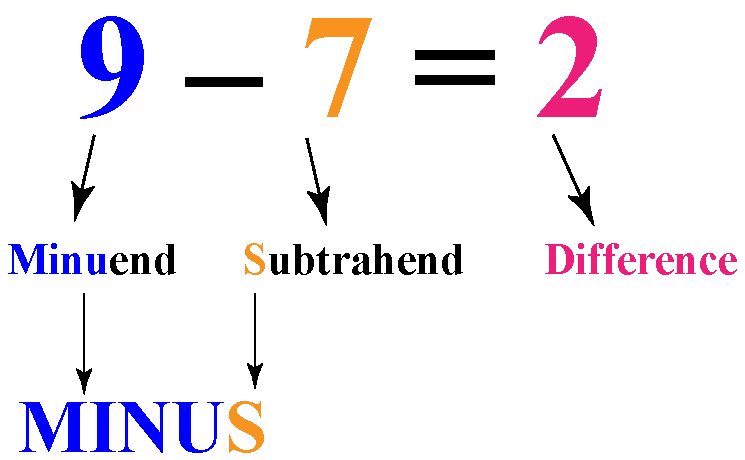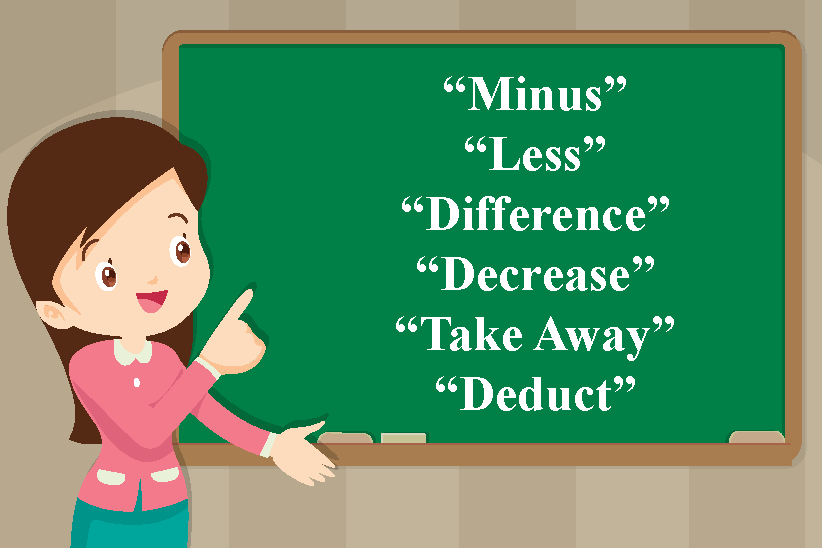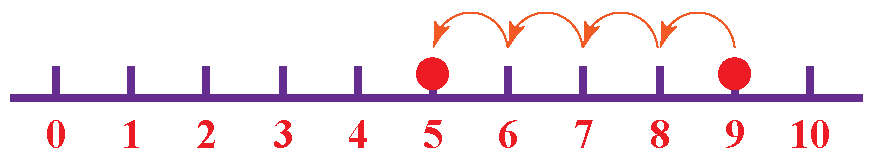# What is Subtraction?

Go back to  'PEMDAS'

Taking away from something is subtraction. Playing with colourful counters, learn how you can subtract numbers in simple steps in this video.

 1 Concept Video on Subtraction 2 Introduction to Subtraction 3 What is Subtraction? 4 Subtraction on Number Line 5 Important Notes on Subtraction 6 Solved Examples on Subtraction 7 Tips and Tricks 8 Subtraction Calculator 9 Thinking out of the Box! 10 Practice Questions on Subtraction 11 Maths Olympiad Sample Papers 12 Frequently Asked Questions (FAQs) 13 Download FREE Worksheets of Subtraction

We at Cuemath believe that Math is a life skill. Our Math Experts focus on the “Why” behind the “What.” Students can explore from a huge range of interactive worksheets, visuals, simulations, practice tests, and more to understand a concept in depth.

Book a FREE trial class today! and experience Cuemath’s LIVE Online Class with your child.

## Concept Video on Subtraction - Cuemath Way

Do you know how to subtract numbers?

If you don't, you can watch this video to learn how to subtract numbers the Cuemath way.

In this Cuemath video, you will learn how to do subtraction by building number combinations using Base Ten Blocks.

## Introduction to Subtraction

When you have a group of things and you subtract few things from it, the group becomes smaller.

For example, you bought $$9$$ cupcakes for your birthday party.

Your friends ate $$7$$ cupcakes.Now you are left with $$2$$ cupcakes.

This can be written as a mathematical equation.This can be read as "nine minus seven equals two".

There are 3 parts to a subtraction problem:

1. Minuend
2. Subtrahend
3. Difference.

## What is Subtraction?

### Subtraction: Definition

In mathematics, subtraction means to take away some things from a group.

In other words, subtraction is the process of removing things from a group.

We use minus sign (-) as the subtraction symbol when one number is subtracted from another number.The terms such as "Minus", "Less", "Difference", "Decrease", "Take Away" and "Deduct" indicates that you need to subtract one number from another.

## Subtraction on Number Line

A number line is a visual aid for you to understand subtraction because it allows you to jump backward and forward on each number.

To understand how this works, let's explore subtraction using a number line.

Let's subtract $$4$$ from $$9$$

We'll start by marking the number $$9$$ on the number line.

When we subtract using a number line, we count by moving one number at a time toward the left.

Since we are subtracting $$4$$ from $$9$$, we will move 4 times to the left.The number on which you land after 4 jumps is the answer. Thus,

$$9-4=5$$

Always remember that when you jump backward from any number, subtraction occurs.

Addition and Subtraction of two-digit numbers
Addition and Subtraction of two-digit numbers
Addition and subtraction of 3-digit numbers
Addition and subtraction of 3-digit numbers
Mental arithmetic
Mental arithmetic
Addition and Subtraction of 4-digit numbers
Mental Arithmetic
Mental Arithmetic
Addition and Subtraction of 4-digit numbers
Kg | Worksheet 5
Kg | Worksheet 6
Addition and Subtraction with numbers up to 20
Kg | Worksheet 3
Addition and Subtraction with numbers up to 20
Kg | Worksheet 4
A Visual Introduction to Subtraction
Kg | Worksheet 2
A Visual Introduction to Subtraction
Kg | Worksheet 1

More Important Topics
Numbers
Algebra
Geometry
Measurement
Money
Data
Trigonometry
Calculus